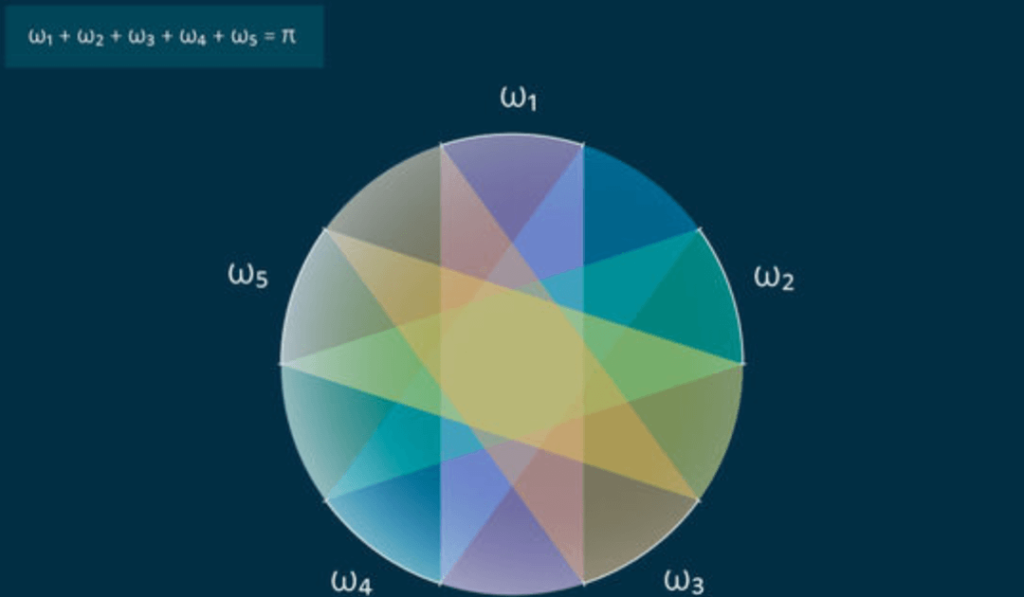# László Fejes Tóth’s theorem proved

{:en}A Chinese-Russian tandem proved László Fejes Tóth’s zone conjecture.

For more than 40 years, mathematicians in the field of discrete and combinatorial geometry were trying to prove the theorem. Finally, Alexandr Polyanskii and Zilin Jiang from the Israel Institute of Technology, managed to do that.

“The solution, when we found it, turned out to be very elegant.. This theorem made us think of another more solid hypothesis of covering a sphere with displaced zones. The zones are obtained by crossing a single sphere with three-dimensional stripes, or planks, not necessarily symmetrical to the centre,” – commented Alexandr Polyanskii .

The mathematicians managed to prove a multidimensional version of the conjecture. A more simplified version was proven by Alfred Tarski (below). According to it, a circle can be fully covered by stripes or planks and their comprehensive widths shall not exceed the circumference of the circle.

This theorem is an integral part of the discrete geometry. For instance, if you have a sphere, it allows calculating how many similarly-sized spheres one can place around it. These mathematical problems often can be applied practically in the domain of IT technologies, physics or chemistry.

The conjecture was proved by contradiction. Hopefully, the proof will contribute to the discrete geometry development and will allow formulating new practical and mathematical questions related to this “theorem of planks”.The sphere is covered with zones, or stripes, of identical width. A minimal comprehensive width of the zones equals π. Each zone has its own unique colour.Illustration of the Alfred Tarski proof. Each fragment of the circle lies between two parallels. The comprehensive width of the stripes equals the diametral line of the circle.

Illustrations provided by the Moscow Institute of Physics and Technology.

{:}{:fr}

{:}{:it}

{:}{:es}

{:}{:pl}

{:}{:br}

{:}

You can support us by buying us a coffee,
Every little bit will go towards creating new and exciting content for you!